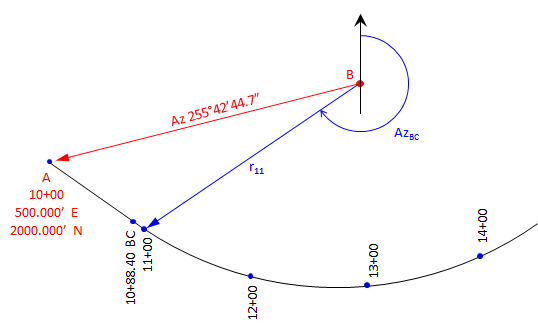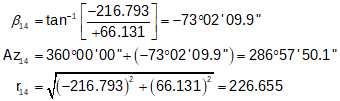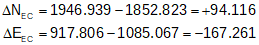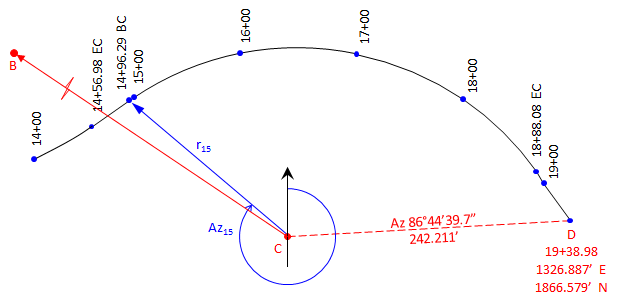### Article IndexThe North and East coordinates of each alignment point are:The Total Station Instrument (TSI) is set up at a known point from which part of the alignment will be staked. Using the coordinates, the distance and direction from the TSI point to each alignment point. is computed. After orienting the TSI on a known basksight (BS) point, an alignment point is staked by turning to its azimuth and measuring out the distance.

Example computations:

 Set TSI at point B. Sight point A with AzB-A=255°42'45" set on the horizontal circle.To stake 10+88.40 BCTurn to horizontal angle reading = 238°25'40" Stake point at 227.17 ft
 To stake 11+00Turn to horizontal angle reading = 235°42'10" Stake point at 222.69 ftTo stake 12+00Turn to horizontal angle reading = 209°17'17" Stake point at 189.79 ftand so on for as many points to be staked from point B.

 Move the TSI to point C; Sight point B with AzC-B = 303°54'34" set on horizontal circle.To stake 14+00Turn to horizontal angle reading = 286°57'50" Stake point at 226.66 ft
 To stake 14+56.98 ECTurn to horizontal angle reading = 300°08'26" Stake point at 193.411 ft
 To stake 14+96.29 BCTurn to horizontal angle reading = 310°48'54" Stake point at 178.41 ft

Depending on sight distances, obstructions, etc, the TSI may need to be relocated a few times to stake all the points.

Since radial stakeout is a series of open traverses, there should be overlap between different TSI setups as field checks. For example, stations 13+00 through 15+00 should be computed from points B and C: they would be staked from one TSI set up and checked from the other.

A complete set of stakeout notes would be:

 TSI at point B; BS point A Point Az, Azimuth r, Distance A - BS 255°42'45" - - 10+88.40 BC 238°25'41" 227.17 11+00 235°42'10" 222.69 12+00 209°17'17" 189.79 13+00 177°53'54" 173.67 14+00 145°21'03" 180.72 14+56.98 EC 128°23'50" 194.30 14+96.29 BC 118°01'09" 209.01 15+00 117°07'24" 210.75 C - Check 123°53'34" - -

 TSI at point C; BS point B Point Az, Azimuth r, Distance B - BS 303°54'34" - - 13+00 286°57'50" 226.66 14+00 299°21'58" 191.22 14+56.98 EC 310°48'54" 178.41 14+96.29 BC 311 58'29 177.50 15+00 345 36'34 161.96 16+00 20 42'10 161.12 17+00 52 04'50" 190.59 18+00 75 23'29" 219.64 18+88.08 EC 78 14'51" 224.09 19+00 86 44'39" 242.21 D - Check 86°44'40" - -